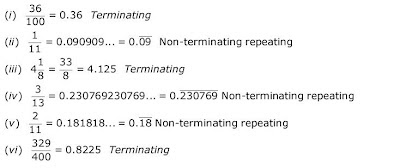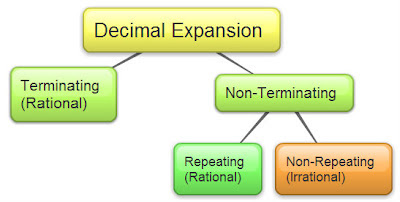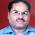## Thursday, 7 June 2012

### Exercise 1.3

Q1. Write the following in decimal form and say what kind of decimal expansion each has:Q3: Express the following in the form p/q, where p and q are integers and q 0.

 (i)(ii)(iii)

Q4: Express 0.99999…in the form p/q. Are you surprised by your answer? With your teacher and classmates discuss why the answer makes sense.

Answer: Let x = 0.9999...
10x = 9.9999...
10x = 9 + 0.9999...   = 9 + x
⇒ 10x -9x = 9
⇒ 9x = 9 ⇒  x = 1
Consider the case 1 - 0.999999 = 0.000001 (negligible difference). This means 0.999... approaches 1. Therefore 1 as an answer is justified.

It also shows that any terminating decimal can be represented as a  non-terminating and recurring decimal expansion with an endless blocks of 9s.
e.g 6 = 5.9999...

Q5: What can the maximum number of digits be in the repeating block of digits in the decimal expansion of 1/17 ? Perform the division to check your answer.

Answer: 1/17 = 0.05882352941176470588235294117647...
i.e. it is 16 repeating digits. Please try the division yourself.

Q6:  Look at several examples of rational numbers in the form p/q (q ≠ 0), where p and q are integers with no common factors other than 1 and having terminating decimal  representations (expansions). Can you guess what property q must satisfy?

Answer: We observe when denominator q is 2, 4, 5, 8, 10..., the decimal expansion is terminating. Consider the following examples:

(i) 7/8 = 0.875. Terminating Decimal. Here, denominator q = 8 ie. 23
(ii) 4/5 = 0.8 (Terminating Decimal). Here, denominator q = 5 ie. 51
(iii) 22/25 = 0.88 (Terminating Decimal). Here, denominator q = 25 ie. 52
(iv) 41/100 = 0.41 (Terminating Decimal). Here, denominator q = 100 = 25 x 4 ie. 52 x  22

By studying similar patterns, we can conclude that for terminating decimal, denominator q has prime factors of the form 2m x  5n where (m = 0,1,2,3... and n = 0,1,2,3,4...)

Q7: Write three numbers whose decimal expansions are non-terminating non-recurring.

1. 0.303003000300003...
2. 0.515115111511115...
3. 0.92092009200092000920000...

Q8: Find three different irrational numbers between the rational numbers 5/7 and 9/11.

Answer: 5/7 = 0.714285714285... = 0.(714285)...
and 9/11 = 0.818181...

Three irrational numbers between  5/7 and 9/11 are:
1. 0.76076007600076...
2. 0.781781178111781111...
3. 0.790790079000790000...

Q9:  Classify the following numbers as rational or irrational:
(i) √(23)        (ii) √(225)    (iii) 0.3796
(iv) 7.478478
(v) 1.101001000100001…

(i) √(23) = 4.79583152331... (non-terminating, non-recurring)

⇒ Irrational Number

(ii) √(225) = 15 = 15/1 = (p/q form, q ≠ 0) ⇒ Rational Number

(iii) 0.3796 Decimal expansion is terminating ⇒ Rational Number

(iv) 7.478478 = 7.(478)... (.478 is recurring pattern). Number is non-terminating but recurring.
⇒ Rational Number

(v) 1.10100100010000 … Decimal expansion is non-terminating, non-repeating.
⇒ Irrational Number

Q11: Express the decimal expansion 0.137454545... as rational number.

Let x =  0.137454545…
⇒  103x  = 137.4545…                                       (I)
and  105x = 13745.4545…                                 (II)

Subtracting I from II,
105x - 103x = 13608
(105 - 103)x = 13608
99000x = 13608
⇒ x = 13608/99000 = 1701/12375           ...(answer)1.great ,,,
i want to share link talking about irrational number too ...

http://www.math-worksheets.co.uk/048-tmd-what-are-irrational-numbers/

2.this is amazing

3.very good solutions is this

4.i think is this better

5.and say to be awesome